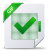# Developing a Rotating Magnetic Field

## Rapport Developing a Rotating Magnetic Field, tutoriel & guide de travaux pratiques en pdf.

### Force and Motion

Before discussing AC motors it is necessary to understand some of the basic terminology associated with motor operation.
Many of these terms are familiar to us in some other context. Later in the course we will see how these terms apply to AC motors.
Force In simple terms, a force is a push or a pull. Force may be caused by electromagnetism, gravity, or a combination of physical means.
Net force Net force is the vector sum of all forces that act on an object, including friction and gravity. When forces are applied in the same direction they are added. For example, if two 10 pound forces are applied in the same direction the net force would be 20 pounds.
If 10 pounds of force is applied in one direction and 5 pounds of force is applied in the opposite direction, the net force would be 5 pounds and the object would move in the direction of the greater force.
If 10 pounds of force is applied equally in both directions, the net force would be zero and the object would not move.
Torque Torque is a twisting or turning force that causes an object to rotate. For example, a force applied to the end of a lever causes a turning effect or torque at the pivot point.
Torque (τ) is the product of force and radius (lever distance). τ = Force x Radius
In the English system torque is measured in pound-feet (lb-ft) or pound-inches (lb-in). For example, if 10 lbs of force is applied to a lever 1 foot long the resulting torque would be 10 lb-ft.
An increase in force or radius would result in a corresponding increase in torque. Increasing the radius to two feet, for example, results in 20 lb-ft of torque.
Speed An object in motion travels a distance in a given time. Speed is the ratio of the distance traveled and the time it takes to travel the distance.
Linear Speed The linear speed of an object determines how long it takes the object to get from point A to point B. Linear speed is usually expressed in a form that combines units of distance divided by units of time such as meters per second (m/s). For example, if the distance between point A and point B were 10 meters, and it took 2 seconds to travel the distance, the speed would be 5 m/s.
Angular (Rotational) Speed The angular speed of a rotating object determines how long it takes a given point on the object to make one complete revolution from its starting point. Angular speed is often expressed in revolutions per minute (RPM). An object that makes ten complete revolutions in one minute, for example, has a speed of 10 RPM.
Acceleration An object can change speed. An increase in speed is called acceleration. Acceleration occurs only when there is a change in the force acting upon the object. An object can also change from a higher to a lower speed. This is known as deceleration (negative acceleration). A rotating object, for example, can accelerate from 10 RPM to 20 RPM, or decelerate from 20 RPM to 10 RPM.
Inertia Mechanical systems are subject to the law of inertia. The law of inertia states that an object will tend to remain in its current state of rest or motion unless acted upon by an external force. This property of resistance to acceleration/deceleration is referred to as the moment of inertia. The English system of measurement is pound-feet squared (lb-ft2 ).
If we look at a continuous roll of paper, for example, we know that when the roll is stopped it would take a certain amount of force to overcome the inertia of the roll to get it rolling. The force required to overcome this inertia can come from a source of energy such as a motor. Once rolling, the paper will continue unwinding until another force acts on it to bring it to a stop.
Friction Any system in motion has losses that drain energy from the system. The law of inertia is still valid, however, because the system will remain in motion at constant speed if energy is added to the system to compensate for the losses. In the previous illustration, for example, these losses include:
• Friction applied to motor and driven equipment bearings
• Wind losses in the motor and driven equipment
• Friction between material on winder and rollers
Work Whenever a force of any kind causes motion, work is accomplished. For example, work is accomplished when an object on a conveyor is moved from one point to another.

Introduction
AC Motors
Force and Motion
AC Motor Construction
Magnetism
Electromagnetism
Developing a Rotating Magnetic Field
Rotor Rotation
Motor Specifications
NEMA Motor Characteristics
Derating Factors
AC Motors and AC Drives
Matching AC Motors to the Load
Enclosures
Mounting
Siemens Motors
Above NEMA Motors
Review Answers
Final ExamTélécharger le cours complet